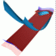Abstract and Contents
Up: 3. The algorithm
Next: 4. Correctness
Previous: 3.3 The case of a hyperbolic fixed point

# 3.4 Mesh adaptation

It is an immediate observation that any nice, equally spaced two-dimensional mesh loses its nice properties under iteration of the nonlinear map f. This has to do with different growth rates, which lead to the accumulation of the mesh on one-dimensional submanifolds as is sketched in Figure 2. Our strategy to avoid this problem is to compute the intersection curves of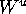with the finitely many leaves of. Along each intersection curve we impose that the mesh has a maximal distance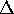between meshpoints. Furthermore, the algorithm is such that corresponding mesh points in neighboring leaves have the same (arclength) distance from the invariant manifold. This is a result of the strategy of adding to the mesh a circle with prescribed (arclength) distance at each step. By default we begin with enough points in M, so that neighboring points in M have maximal distance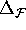. (It may be useful during computations to allow the distancebetween mesh points in one leaf to be different from the distancebetween mesh points in neighboring leaves.)

In other words, by definition the mesh is uniform in the beginning of the computation, so that each edge of the triangulation has a maximal length of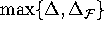. The only problem is that later in the computation neighboring points in different leaves may have a distance larger than. The solution is to add leaves during the computation to guarantee that the mesh stays uniform.

Up: 3. The algorithm
Next: 4. Correctness
Previous: 3.3 The case of a hyperbolic fixed pointAbstract and Contents

Written by: Bernd Krauskopf & Hinke Osinga
Created: May 27 1997 --- Last modified: Fri May 30 19:52:23 1997